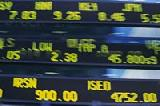Financial Terms Moving-averages chart

# Definition of Moving-averages chart## Moving-averages chart

A financial chart that plots leading and lagging
moving averages for prices or values of an asset.

# Related Terms:

## Bollinger band chart

A financial chart that plots actual asset data along
with three other bands of data: the upper band is two standard deviations
above a user-specified moving average; the lower band is two standard
deviations below that moving average; and the middle band is the moving
average itself.

## break-even chart

a graph that depicts the relationships among revenues, variable costs, fixed costs, and profits (or losses)

## Candlestick chart

A financial chart usually used to plot the high, low, open,
and close price of a security over time. The body of the “candle” is the region
between the open and close price of the security. Thin vertical lines extend up
to the high and down to the low, respectively. If the open price is greater than
the close price, the body is empty. If the close price is greater than the open

## Chart of accounts

A listing of all accounts used in the general ledger, usually sorted in
order of account number.

## Chartists

Related: technical analysts.

## control chart

a graphical presentation of the results of a
specified activity; it indicates the upper and lower control
limits and those results that are out of control

## Corporate charter

A legal document creating a corporation.## High-low-close chart

A financial chart usually used to plot the high, low,
open, and close price of a security over time. Plots are vertical lines whose top
is the high, bottom is the low, open is a short horizontal tick to the left, and
close is a short horizontal tick to the right.

## Moving average

Used in charts and technical analysis, the average of security or commodity prices
constructed in a period as short as a few days or as Long as several years and showing trends for the latest
interval. As each new variable is included in calculating the average, the last variable of the series is deleted.

## Moving average

parametrically determined prices over some time period.

## Moving average inventory method

An inventory costing methodology that calls for the re-calculation of the average cost of all parts in stock after every purchase.
Therefore, the moving average is the cost of all units subsequent to the latest purchase,
divided by their total cost.

## organization chart

a depiction of the functions, divisions,
and positions of the people/jobs in a company and how
they are related; it also indicates the lines of authority and
responsibility

## Point and figure chart

A price-only chart that takes into account only whole integer changes in price, i.e., a
2-point change. Point and figure charting disregards the element of time and is solely used to record changes
in price.

## Point and figure chart

A financial chart usually used to plot asset price data.
Upward price movements are plotted as X’s and downward price movements
are plotted as O’s.

## Simple moving average

The mean, calculated at any time over a past period of fixed length.

## Slow-moving item

An inventory item having a slower rate of turnover than the
average turnover for the entire inventory.

## value chart

a visual representation indicating the valueadded
and non-value-added activities and time spent in
those activities from the beginning to the end of a process